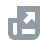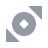Kup kryptowaluty
Zapłać przez
Rynki
NFT
Feed
USD

# FAQFunkcje KontaTutorialBinance Fan TokenBinance EarnWpłaty/Wypłaty KryptoKup Krypto (Fiat/P2P)Handel Spot i MarginKrypto DerywatyFinanseAPIBezpieczeństwoWarunki użytkowaniaBinance ConvertNFTVIP
Strona Główna
Centrum Wsparcia
FAQ
Krypto Derywaty
Kontrakty Futures
Przewodnik po Futures
How to Calculate Return on Investment (ROI)

# How to Calculate Return on Investment (ROI)

2020-12-03 10:49
Return on Investment (ROI) is a ratio or percentage value that reflects the profitability or efficiency of a certain trade or investment. It is a simple-to-use tool that can generate an absolute ratio (e.g. 0.35) or a value in percentage (e.g. 35%). As such, ROI can also be used when comparing different types of investments or multiple trading operations.
Specifically, ROI evaluates the return on an investment in relation to its purchasing cost. This means that the calculation of ROI is simply the return (net profit) divided by the total acquisition costs (net cost). The result may then be multiplied by 100 to get the percentage value.
Naturally, a high ROI value indicates that the investment was profitable, while a negative ROI means the return was lower than the costs. The calculation of ROI is based on the following equation:
ROI = (Current Value - Total Cost) / Total Cost
Alternatively, it may also be written as:
ROI = Net Profit / Net Cost
As an example, imagine that Alice bought 100 BNB for 1,000 US dollars - paying 10 dollars each. If the current price of BNB is 19 dollars, Alice would have a ROI of 0.90 or 90%.
ROI is widely used in both traditional and cryptocurrency markets. However, it has some limitations. For instance, Alice may use the ROI formula when comparing two different trades. However, the equation does not take the time into account.
This means that in some situations, one investment may seem more profitable than the other when, in reality, its efficiency was lower because it required a much longer period. So, if Alice’s first trade had a 90% ROI but took 12 months to happen, it would be less efficient than a second trade that had, for example, a 70% ROI in 6 months.
ROI is a way to measure an investment's performance. Additionally, it's also a great way to compare the profitability of different investments. Naturally, an investment with a higher ROI is better than an investment with a lower (or negative) ROI. Curious how to measure this for your own portfolio? Read more on Binance Academy: How to Calculate Return on Investment (ROI).